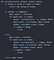Minimax

The algorithm is used for optimal decision-making in game theory and artificial intelligence. In the minimax algorithm, the entire game tree is searched and the algorithm finds the best possible move by working from the bottom up and assuming both players are playing perfectly.

The computer is making a move at the root A and is choosing between nodes B and C. The leaf nodes are values that correspond to outcomes of the game. The goal for the computer is for the game to end in the outcome with the highest possible value, while the goal for the human is the opposite.

The main disadvantage with this procedure is that this algorithm requires the computer to traverse the entire tree, which can require a lot of time and computing power. The vanilla algorithm’s performance can be heavily improved by using alpha-beta pruning. It stops evaluating a move when it makes sure that it’s worse than previously examined move — such moves need not to be evaluated further.

Alpha-Beta Pruning

Alpha-beta pruning involves two threshold parameters alpha (α) and beta (β) which are used to keep track of the best score either player can achieve while walking the tree. Alpha is the best choice for player MAX. We want to get the highest possible value here. Beta is the best choice for MIN, and it has to be the lowest possible value here. The main concept is to maintain two values through whole search.

This method returns the same moves as the standard one, but it prunes all the nodes that are possibly not affecting the final decision.The minimax algorithm with alpha-beta pruning in pseudocode for Tic-Tac-Toe game

Let’s make above algorithm clear with an example.

Step 1: The initial call starts from A. The value of alpha (α) here is -∞ and the value of beta (β) is +∞. These values are passed down to node B where again α = -∞ and β = +∞, and node B passes the same value to its child D.

Step 2: Since it is the move of the player Max, we will choose the maximum of all the utilities. For this case, we have to evaluate max(2, 3). So 3 will be the value of α at node D.

Step 3: Now the algorithm backtracks to node B, since this is a turn of Min, i.e. min(∞, 3), hence node B now has α = -∞ and β = 3.

Step 4: The algorithm traverses the next child of Node B which is node E. At E, the values of (α, β) are not (-∞, ∞) but instead the values (-∞, 3) passed down from node B. Because it’s Max’s turn, α will compare its current value with 5 and take the maximum max(-∞, 5). Hence α = 5 and β = 3 and α >= β at node E, the right child of E will be pruned.

The algorithm will not traverse it no matter what the value of E‘s right child is. We never had to look at it because the minimizer was guaranteed a value of 3 or lesser.

At node B, β = min( 3, 5) which is 3, so the value of node B is 3.

Step 5: The algorithm again traces back the tree to its root. At node A, the value of alpha will be changed to the maximum value of max(-∞, 3). So now A has α = -∞ and β= +∞, and these two values are passed to the right child of A.

Step 6: At node C, α = 3 and β = +∞, and the same values will be passed on to node F. At F, α = 3 and β = +∞. It looks at its left child and takes the maximum value between its current α and 0 and then compares it with the right child. The alpha value will remain 3 at node F, but its value is max(0, 1) which is 1.

Step 7: Node C will take 1 from F as its β value. Now C has α=3 and β= 1, and again it satisfies the condition α>=β, so the right child of C will be pruned, and the algorithm will not traverse the entire subtree G.

Step 8: C now returns a value of 2 to A. Therefore the best value at A is max( 5, 2) which is a 5. The picture above is the final game tree which shows the nodes that are computed and nodes that have never computed. Hence the optimal value for the maximizer is 3 for this example.

Let’s see the difference between the vanilla minimax algorithm and the minimax algorithm with alpha-beta pruning in Tic-Tac-Toe game: